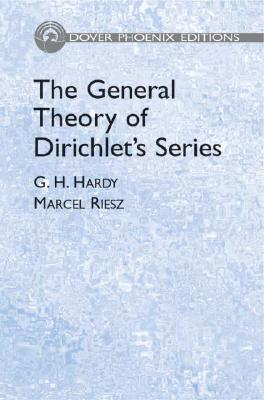### The General Theory of Dirichlet's Series (Hardcover)

Dover Publications, 9780486446578, 96pp.

Publication Date: November 1, 2005

List Price: 30.00*
* Individual store prices may vary.

#### Description

This classic work explains the theory and formulas behind Dirichlet's series and offers the first systematic account of Riesz's theory of the summation of series by typical means. Its authors rank among the most distinguished mathematicians of the twentieth century: G. H. Hardy is famous for his achievements in number theory and mathematical analysis, and Marcel Riesz's interests ranged from functional analysis to partial differential equations, mathematical physics, number theory, and algebra.
Following an introduction, the authors proceed to a discussion of the elementary theory of the convergence of Dirichlet's series, followed by a look at the formula for the sum of the coefficients of a Dirichlet's series in terms of the order of the function represented by the series. They continue with an examination of the summation of series by typical means and of general arithmetic theorems concerning typical means. After a survey of Abelian and Tauberian theorems and of further developments of the theory of functions represented by Dirichlet's series, the text concludes with an exploration of the multiplication of Dirichlet's series.